# Online Civil Engineering Test - Previous GATE Exam Papers Test 3Loading Test...

Instruction:

• This is a FREE online test. DO NOT pay money to anyone to attend this test.
• Total number of questions : 20.
• Time alloted : 30 minutes.
• Each question carry 1 mark, no negative marks.
• DO NOT refresh the page.
• All the best :-).

1.

A water treatment plant is required to process 28800 m3/d of raw water (density = 1000 kg/m3, kinematic viscosity = 10-6 m2/s). The rapid mixing tank imparts tank a velocity gradient of 900s-1 to blend 35 mg/l of alum with the flow for a detention time of 2 minutes. The power input (W) required for rapid mixing is

A.
 32.4B.
 36C.
 324D.
 324002.

The vertical stress at depth z, directly below the point load p is (k is a constant)

A.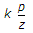B.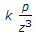C.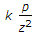D.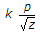3.

The drop manholes are provided in a sewerage system when there is

A.
 change in alignment of sewer lineB.
 change in size of sewersC.
 change in the elevation of ground levelD.
 change from gravity system to pressure system4.

Presence of excess nitrates in river water indicates :

A.
 recent pollution of water with sewageB.
 past pollution of water with sewageC.
 immediate pollution of water with sewageD.
 no pollution of water with sewage5.

The superelevation needed for a vehicle travelling at a speed of 60 kmph on a curve of radius 128 m on a surface with a coefficient of friction of 0.15 is :

A.
 0.71B.
 0.81C.
 0.91D.
 0.616.

Reaction at support b of the structure is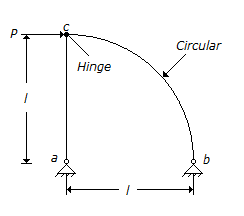A.
 PB.
 P2C.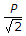D.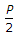7.

A vertical rod PQ of length L is fixed at its top end P and has a flange fixed to the bottom end Q. A weight W is dropped vertically from a height h(< L) on to the flange. The axial stress in the rod can be reduced by

A.
 increasing the length of the rodB.
 decreasing the length of the rodC.
 decreasing the area of cross-section of the rodD.
 increasing the modulus of elasticity of the material8.

A linear relationship is observed between speed and density on a certain section of a highway. The free flow speed is observed to be 80 km per hour and the jam density is estimated as 100 vehicles per km length. Based on the above relationship, the maximum flow expected on this section and the speed at the maximum flow will respectively be

A.
 8000 vehicles per hour and 80 km per hourB.
 8000 vehicles per hour and 25 km per hourC.
 2000 vehicles per hour and 80 km per hourD.
 2000 vehicles per hour and 40 km per hour9.

The total length (in km) of the existing National Highways in India is in the range of

A.
 15, 000 to 25, 000B.
 25, 000 to 35, 000C.
 35, 000 to 45, 000D.
 45, 000 to 55, 00010.

A point load of 700 kN is applied on the surface of thick layer of clay. Using Boussinesq's elastic analysis, the estimated vertical stress (σv) at a depth of 2 m and a radial distance of 1.0 m from the point of application of the load, is :

A.
 47.5 kPaB.
 47.6 kPaC.
 47.7 kPaD.
 47.8 kPa11.

A propped cantilever beam of span L, is loaded with uniformly distributed load of intensity w/unit length, all through the span. Bending moment at the fixed end is,

A.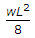B.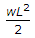C.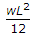D.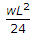12.

The dimensions for the flexural rigidity of a beam element in mass (M), length (L) and time (T) is given by

A.
 MT-2B.
 ML3T-2C.
 ML-1T-2D.
 M-1T213.

The time for a clay layer to achieve 90% consolidation is 15 years. The time required to achieve 90% consolidation; if the layer were twice as thick, 3 times forr permeable and 4 times more compressible would be :

A.
 70 yearsB.
 75 yearsC.
 80 yearsD.
 85 years14.

An outlet irrigates an area of 20 ha. The discharge (l/s) required at this outlet to meet the evapotranspiration requirement of 20 mm occurring uniformly in 20 days neglecting other field losses is

A.
 2.52B.
 2.31C.
 2.01D.
 1.5215.

The consistency of a saturated cohesive soil is affected by

A.
 water contentB.
 particle size distributionC.
 density indexD.
 coefficient of permeability16.

In a river, discharge is 173 m3/s; water surface slope is 1 in 6000; and stage at the gauge station is 10.0 m. If during a flood, the stage at the gauge station is same and the water surface slope is 1 in 2000, the flood discharge in m3/s, is approximately

A.
 371B.
 100C.
 519D.
 30017.

The function of ballast in railaway tracks is to

A.
 facilitate drainageB.
 serve as an elastic support for the track structureC.
 provide the necessary resilience against the dynamic effect of the loadsD.
 all the above18.

A foundation is considered as shallow if its depth is :

A.
 less than 1 metreB.
 greater than its widthC.
 equal to or less than its widthD.
 greater than 1 metre19.

The maximum allowable compressive stress corresponding to lateral buckling in a discretely laterally supported symmetrical I beam does not depend upon :

A.
 the modulus of elasticityB.
 the radius of gyration about the minor axisC.
 the span length of the beamD.
 the ratio of overall depth of thickness of the flange20.

The soils most susceptible to liquefaction are :

A.
 saturated dense sandsB.
 saturated fine and medium sands of uniform particle sizeC.
 saturated clays of uniform sizeD.
 saturated gravels and cobbles